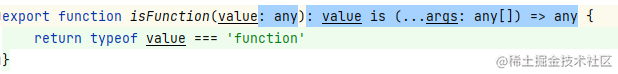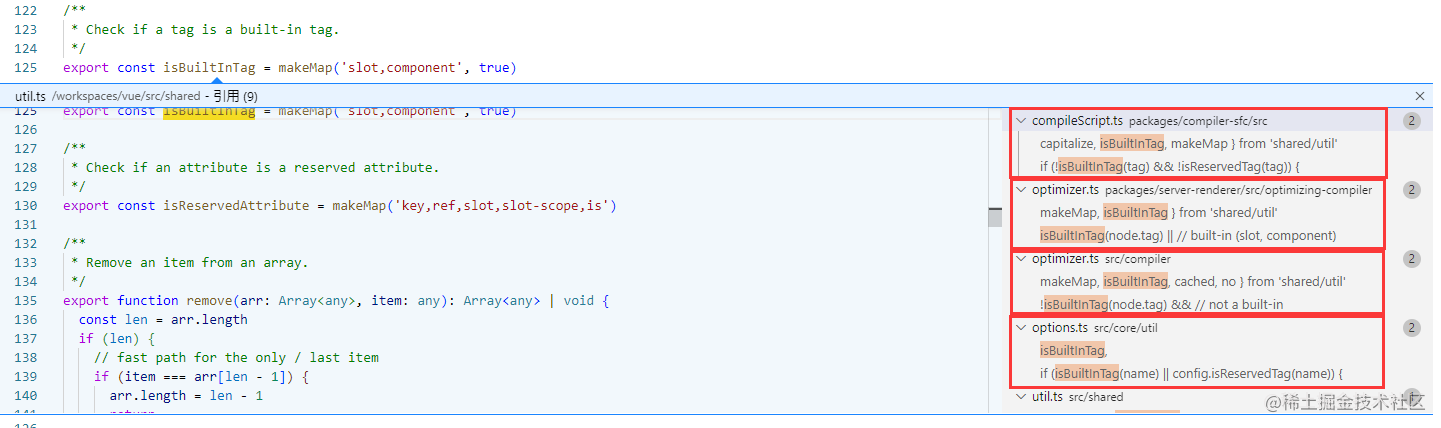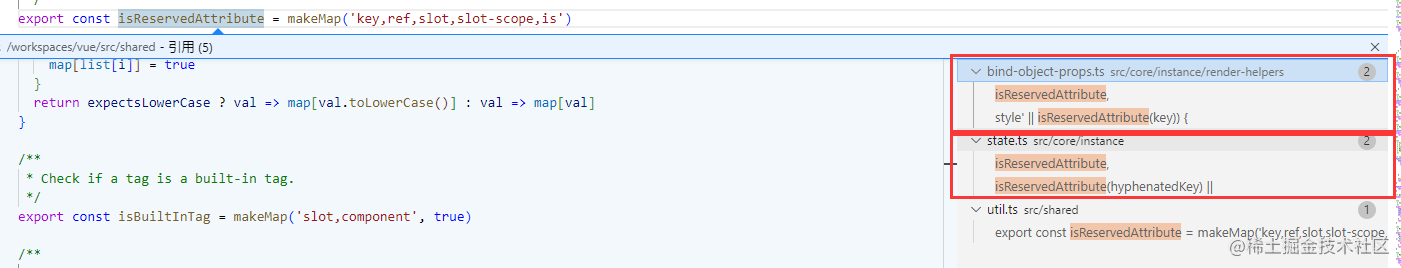# 【源码共读】Vue2源码 shared 模块中的36个实用工具函数分析``````export function isFunction(value) {
return typeof value === 'function'
}

``````var a: <T>(a: T) => T = function<E>(a: E): E {
return a
}

``````var a = function(a) {
return a
}

## 所有的工具函数

• `emptyObject`: 被冻结的空对象
• `isArray`: es6的`Array.isArray`方法
• `isUndef`: 判断是否是`undefined`或者`null`
• `isDef`: 判断是否不是`undefined`并且不是`null`
• `isTrue`: 判断是否是`true`
• `isFalse`: 判断是否是`false`
• `isPrimitive`: 判断是否是原始类型
• `isFunction`: 判断是否是函数
• `isObject`: 判断是否是对象
• `toRawType`: 获取对象的原始类型
• `isPlainObject`: 判断是否是纯对象
• `isRegExp`: 判断是否是正则
• `isValidArrayIndex`: 判断是否是有效的数组索引
• `isPromise`: 判断是否是`Promise`对象
• `toString`: 将值转换为实际呈现的字符串
• `toNumber`: 将值转换为数字
• `makeMap`: 生成一个包含指定字符串的对象
• `isBuiltInTag`: 判断是否是内置标签
• `isReservedAttribute`: 判断是否是保留属性
• `remove`: 从数组中移除指定项
• `hasOwn`: 判断对象是否有指定属性
• `cached`: 缓存函数
• `camelize`: 将字符串转换为驼峰格式
• `capitalize`: 将字符串首字母大写
• `hyphenate`: 将字符串转换为连字符格式
• `bind`: 将函数绑定到指定的上下文
• `toArray`: 将类数组转换为数组
• `extend`: 将源对象的属性拷贝到目标对象
• `toObject`: 将数组转换为对象
• `noop`: 空函数
• `no`: 返回`false`
• `identity`: 返回传入的值
• `genStaticKeys`: 生成静态键
• `looseEqual`: 比较两个值是否相等
• `looseIndexOf`: 获取指定值在数组中的索引
• `once`: 保证函数只执行一次
• `hasChanged`: 判断两个值是否不相等

### emptyObject

``````export const emptyObject = Object.freeze({})

### isArray

``````export const isArray = Array.isArray

### isUndef

``````export function isUndef(v) {
return v === undefined || v === null
}

### isDef

``````export function isDef(v) {
return v !== undefined && v !== null
}

### isTrue

``````export function isTrue(v) {
return v === true
}

### isFalse

``````export function isFalse(v) {
return v === false
}

### isPrimitive

``````export function isPrimitive(value) {
return (
typeof value === 'string' ||
typeof value === 'number' ||
// \$flow-disable-line
typeof value === 'symbol' ||
typeof value === 'boolean'
)
}

### isFunction

``````export function isFunction(value) {
return typeof value === 'function'
}

### isObject

``````export function isObject(obj) {
return obj !== null && typeof obj === 'object'
}

### toRawType

``````const _toString = Object.prototype.toString

export function toRawType(value) {
return _toString.call(value).slice(8, -1)
}

### isPlainObject

``````export function isPlainObject(obj) {
return _toString.call(obj) === '[object Object]'
}

### isRegExp

``````export function isRegExp(v) {
return _toString.call(v) === '[object RegExp]'
}

### isValidArrayIndex

``````export function isValidArrayIndex(val) {
const n = parseFloat(String(val))
return n >= 0 && Math.floor(n) === n && isFinite(val)
}

1. 先将值做`String`转换
2. 然后使用`parseFloat`转换为数字，这里使用`parseFloat`而不是`parseInt`，因为`parseInt`会将`3.14`转换为`3`，而`parseFloat`会保留小数点后面的值；
3. 判断值是否大于等于`0`，因为数组索引不能为负数；
4. 判断值是否是一个整数，这里使用`Math.floor`向下取整，然后和原值做严格相等，因为`3.14``3`是不相等的；
5. 判断值是否是有限的

### isPromise

``````export function isPromise(val) {
return (
isDef(val) &&
typeof val.then === 'function' &&
typeof val.catch === 'function'
)
}

### toString

``````function toString(val) {
return val == null
? ''
: Array.isArray(val) || (isPlainObject(val) && val.toString === _toString)
? JSON.stringify(val, null, 2)
: String(val)
}

1. 如果值是`null`或者`undefined`，返回空字符串；
2. 如果值是数组或者是一个纯对象，且没有重写`toString`方法，使用`JSON.stringify`转换为字符串，这里使用`JSON.stringify`的第二个参数为`null`，第三个参数为`2`，表示缩进为`2`个空格；
3. 如果都不是，使用`String`转换为字符串。

### toNumber

``````function toNumber(val) {
const n = parseFloat(val)
return isNaN(n) ? val : n
}

### makeMap

``````export function makeMap(str, expectsLowerCase) {
const map = Object.create(null)
const list = str.split(',')
for (let i = 0; i < list.length; i++) {
map[list[i]] = true
}
return expectsLowerCase ? val => map[val.toLowerCase()] : val => map[val]
}

### isBuiltInTag

``````export const isBuiltInTag = makeMap('slot,component', true)### isReservedAttribute

``````export const isReservedAttribute = makeMap('key,ref,slot,slot-scope,is')### remove

``````export function remove(arr, item) {
const len = arr.length
if (len) {
// fast path for the only / last item
if (item === arr[len - 1]) {
arr.length = len - 1
return
}
const index = arr.indexOf(item)
if (index > -1) {
return arr.splice(index, 1)
}
}
}

### hasOwn

``````export const hasOwnProperty = Object.prototype.hasOwnProperty
export function hasOwn(obj, key) {
return hasOwnProperty.call(obj, key)
}

### cached

``````export function cached(fn) {
const cache = Object.create(null)
return function cachedFn(str: string) {
const hit = cache[str]
return hit || (cache[str] = fn(str))
}
}

### camelize

``````const camelizeRE = /-(\w)/g
export const camelize = cached((str) => {
return str.replace(camelizeRE, (_, c) => (c ? c.toUpperCase() : ''))
})

### capitalize

``````export const capitalize = cached((str) => {
return str.charAt(0).toUpperCase() + str.slice(1)
})

### hyphenate

``````const hyphenateRE = /\B([A-Z])/g
export const hyphenate = cached((str) => {
return str.replace(hyphenateRE, '-\$1').toLowerCase()
})

### bind

``````/**
* Simple bind polyfill for environments that do not support it,
* e.g., PhantomJS 1.x. Technically, we don't need this anymore
* since native bind is now performant enough in most browsers.
* But removing it would mean breaking code that was able to run in
* PhantomJS 1.x, so this must be kept for backward compatibility.
*/

/* istanbul ignore next */
function polyfillBind(fn, ctx) {
function boundFn(a) {
const l = arguments.length
return l
? l > 1
? fn.apply(ctx, arguments)
: fn.call(ctx, a)
: fn.call(ctx)
}

boundFn._length = fn.length
return boundFn
}

function nativeBind(fn, ctx) {
return fn.bind(ctx)
}

// @ts-expect-error bind cannot be `undefined`
export const bind = Function.prototype.bind ? nativeBind : polyfillBind

### toArray

``````export function toArray(list, start) {
start = start || 0
let i = list.length - start
const ret = new Array(i)
while (i--) {
ret[i] = list[i + start]
}
return ret
}

es6中有`Array.from`方法，可以将类数组转换为数组，扩展阅读：Array.from()

### extend

``````export function extend(to, _from) {
for (const key in _from) {
to[key] = _from[key]
}
}

### toObject

``````export function toObject(arr) {
const res = {}
for (let i = 0; i < arr.length; i++) {
if (arr[i]) {
extend(res, arr[i])
}
}
return res
}

es6中有`Object.assign`方法，可以将对象合并为一个对象，扩展阅读：Object.assign()

### noop

``````export function noop(a, b, c) {}

### no

``````export const no = (a, b, c) => false

### identity

``````export const identity = _ => _

### genStaticKeys

``````/**
* @param modules Array<{ staticKeys?: string[] }
* @return {*}
*/
export function genStaticKeys(modules) {
return modules.reduce((keys, m) => {
return keys.concat(m.staticKeys || [])
}, []).join(',')
}

### looseEqual

``````export function looseEqual(a, b) {
if (a === b) return true
const isObjectA = isObject(a)
const isObjectB = isObject(b)
if (isObjectA && isObjectB) {
try {
const isArrayA = Array.isArray(a)
const isArrayB = Array.isArray(b)
if (isArrayA && isArrayB) {
return (
a.length === b.length &&
a.every((e, i) => {
return looseEqual(e, b[i])
})
)
} else if (a instanceof Date && b instanceof Date) {
return a.getTime() === b.getTime()
} else if (!isArrayA && !isArrayB) {
const keysA = Object.keys(a)
const keysB = Object.keys(b)
return (
keysA.length === keysB.length &&
keysA.every(key => {
return looseEqual(a[key], b[key])
})
)
} else {
/* istanbul ignore next */
return false
}
} catch (e) {
/* istanbul ignore next */
return false
}
} else if (!isObjectA && !isObjectB) {
return String(a) === String(b)
} else {
return false
}
}

``````function looseEqual(a, b) {
if (a === b) return true
return equal(a, b)
}

function equal(obj1, obj2) {
const isObjectA = isObject(a)
const isObjectB = isObject(b)
if (isObjectA && isObjectB) {
try {
return arrayEqual(obj1, obj2) || dateEqual(obj1, obj2) || objectEqual(obj1, obj2)
} catch (e) {
/* istanbul ignore next */
return false
}
} else if (!isObjectA && !isObjectB) {
return String(a) === String(b)
} else {
return false
}
}

function arrayEqual(arr1, arr2) {
const isArrayA = Array.isArray(a)
const isArrayB = Array.isArray(b)
if (isArrayA && isArrayB) {
return (
a.length === b.length &&
a.every((e, i) => {
return looseEqual(e, b[i])
})
)
}
return false
}

function dateEqual(date1, date2) {
if (a instanceof Date && b instanceof Date) {
return a.getTime() === b.getTime()
}
return false
}

function objectEqual(obj1, obj2) {
const keysA = Object.keys(obj1)
const keysB = Object.keys(obj2)
return (
keysA.length === keysB.length &&
keysA.every(key => {
return looseEqual(a[key], b[key])
})
)
}

1. 如果两个值是相等的，直接返回`true`，这里的相等是指`===`，也就是说，如果两个值是引用类型，那么只有当两个值的引用地址相同时才会返回`true`
2. 如果两个值都是`Array`，那么判断两个数组的长度是否相等，如果相等，那么再判断两个数组中的每个元素是否相等，如果相等，递归到`looseEqual`函数中；
3. 如果两个值都是`Date`，那么判断两个`Date`对象的时间戳是否相等；
4. 如果两个值都是`Object`，那么判断两个对象的键值对个数是否相等，如果相等，递归到`looseEqual`函数中；

``````graph TB
A[looseEqual] --> B[a==b]
B -->|是| C[return true]
B -->|否| D[a b 都是数组]
D -->|是| E[判断两个数组的长度是否相等]
E -->|否| G[a b 都是日期]
E -->|是| F[判断两个数组中的每个元素是否相等]
F --> A
G -->|否| H[a b 都是对象]
G -->|是| I[判断两个日期的时间戳是否相等]
I -->|否| J[return false]
I -->|是| K[return true]
H -->|否| L[a b 都不是对象]
L -->|否| M[return false]
L -->|是| N[判断两个对象的键值对个数是否相等]
N -->|否| O[return false]
N -->|是| P[判断两个对象中的每个键值对是否相等]
P --> A
``````

### looseIndexOf

``````function looseIndexOf(arr, val) {
for (let i = 0; i < arr.length; i++) {
if (looseEqual(arr[i], val)) return i
}
return -1
}

### once

``````function once(fn) {
let called = false
return function() {
if (!called) {
called = true
fn.apply(this, arguments)
}
}
}

### hasChanged

``````export function hasChanged(x, y) {
if (x === y) {
return x === 0 && 1 / x !== 1 / y
} else {
return x === x || y === y
}
}